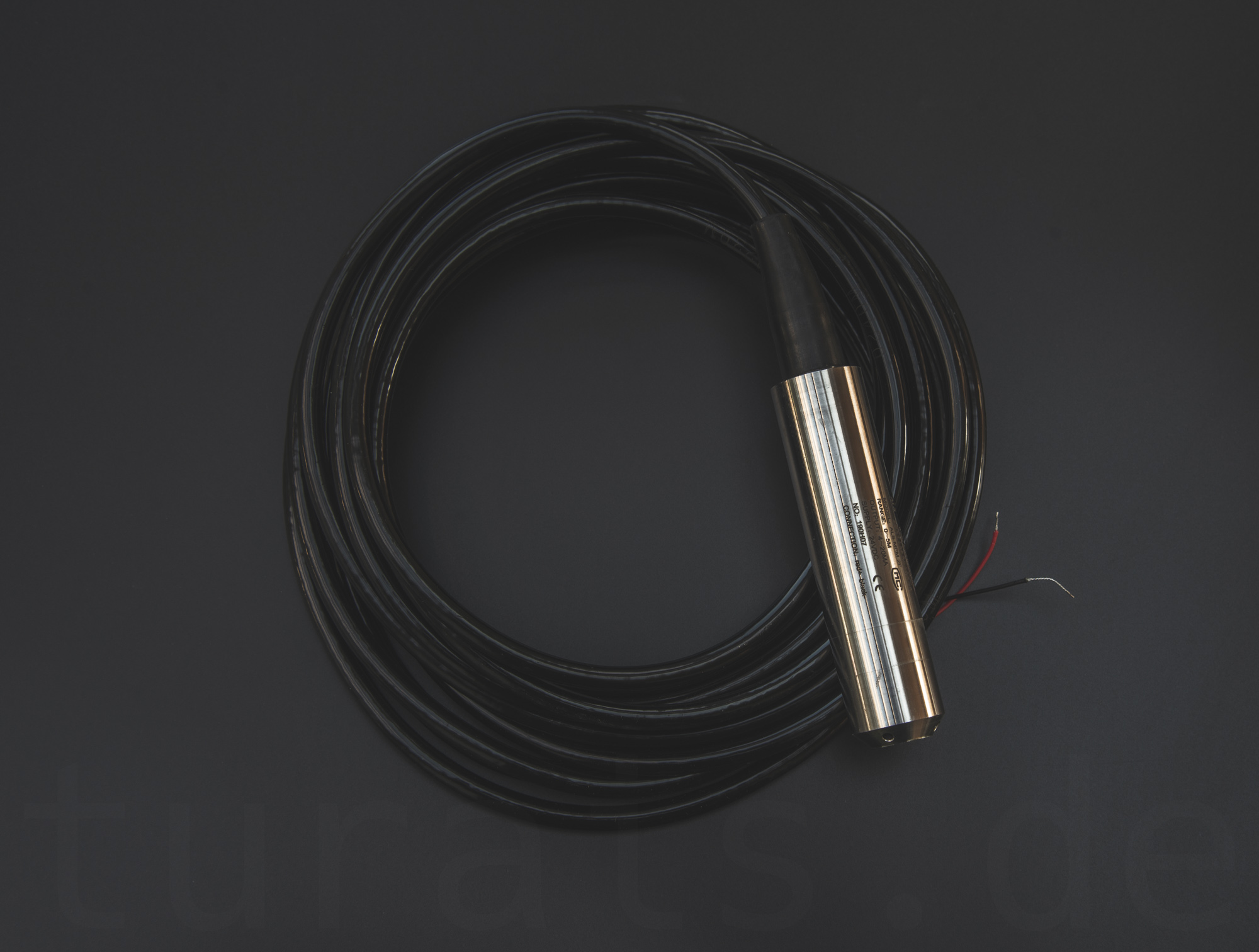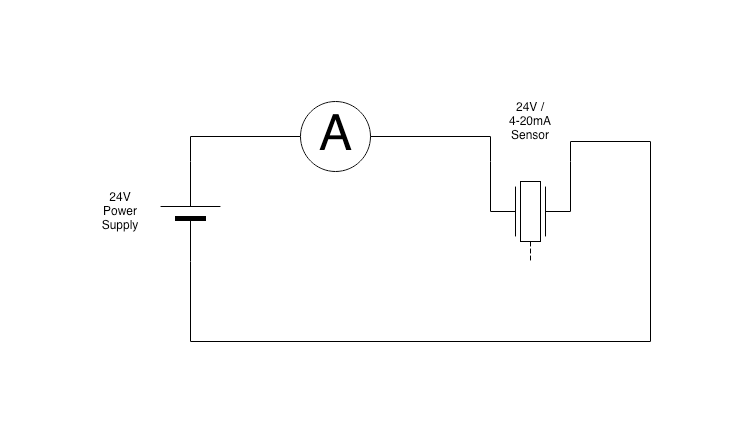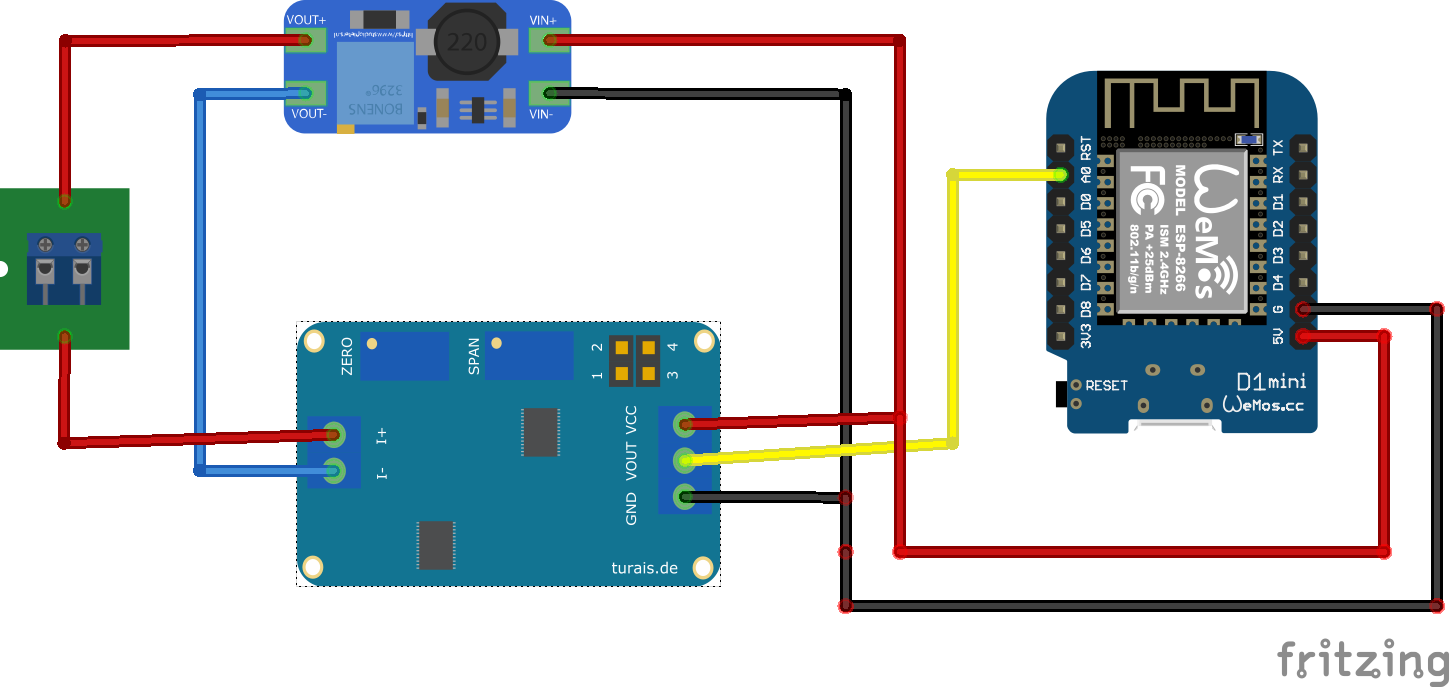# TL231 - 24V 4-20mA Water Level Sensor

The Liquid Water Level Sensor is based on an underwater pressure sensor. With this sensor you can measure distance to the water surface based on the water pressure.

## Overview

The TL231 Water Level Sensor is based on a pressure sensor which measures relative water pressure. I also wrote an Blog Post about this sensor. Blog Post### Pascal’s Law

With the measured pressure at our sensor we can determine the height of water above the sensor.

$$\Delta p = \rho \cdot g \cdot \Delta h$$

where: $p$ is the hydrostatic pressure in Pascal. $g$ is the gravity of earth. Usually around $9.8 \frac{m}{s^2}.$. $\rho$ is just the fluid density, in $1 \frac{kg}{m^3}$. $\Delta h$ is the variable we are interested in. This is the height of water above the sensor. So:

$$\Delta h = \frac{\Delta p}{\rho \cdot g}$$

We can say that $g$ is constant. (It isn’t, but it will not change a lot in our 5 meter measuring range). Also we know the fluid density of water is constant, because water is incompressible. It’s: $1000 \frac{kg}{m^3}$.

Therefore we have a linearity between the change in height and the change in pressure. This means, that whenever the water above the sensor changes, the pressure changes. Therefore we can relate that the pressure change, which we can measure, is a change in the height of the water above the sensor.

$$\Delta h \sim \Delta p$$

### Interpreting Height Change

We now know that a change in pressure is a change in the height of water above the sensor. The Sensor gives you an output of current in relation to the pressure. The current changes from 4mA to 20mA. Where 4mA represents the pressure you’ll have with no water above the sensor, and 20mA with the maximum water level for that sensor. Check that up with your specific sensor. In most cases these kind of sensors have a measuring range from 0 to 5 meters.

### 4-20mA

The 4-20mA Liquid Level Sensor uses a so called current loop. There are active sensors, and passive ones. The difference is the way you power your sensor. Active ones mostly have dedicated wires for power supply, where the passive ones mostly only have 2-wires. The principle is that you put your passive sensor in series to the 24V power supply. A change in height will therefore result in an change in current, the easiest way to get some readings is with an Multimeter.

## Connection

#### Basic Connection DiagramBasic connection of a 4-20mA SensorExample

For the above example you need:

Material needed Alternative/Explanation
Wemos D1 Mini any other Arduino
24V Boost Converter another 24V Power Supply
HW-685 Voltage Divider, DFRobot Converter
Liquid Level Sensor TL231, TL-231, TL136, ASL-MP-2F, …
Constant Voltage Power Supply for your Arduino

## Usage

To use this sensor you need a way to measure current. The sensor changes its current output in relation to the water level above the sensor. If you used the above example and connected everything like in the example, your sensor should give you first results if dipped into water.

Of course there are many more usages, for example with an PLC.

## Code

Check out the HW-685 example. You basically read a value with your ADC. On the Arduino-Platform this is done via

analogRead(PIN_NUM);